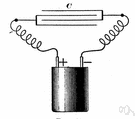# ohmic resistance

Also found in: Thesaurus, Encyclopedia.
ThesaurusAntonymsRelated WordsSynonymsLegend:
 Noun 1ohmic resistance - a material's opposition to the flow of electric current; measured in ohmselectrical phenomenon - a physical phenomenon involving electricityohmage - the ohmic resistance of a conductor
Based on WordNet 3.0, Farlex clipart collection. © 2003-2012 Princeton University, Farlex Inc.
References in periodicals archive ?
Every electrical conductor comprises capacitance, an inductance, and a frequency-dependent ohmic resistance. With increasing frequencies, these electrical characteristics will influence and distort the signal.
where S, P, l are, respectively, the section, [cm.sup.2], perimeter, cm, length, m; [R.sub.0] is the ohmic resistance of steel conductor to direct current, [OMEGA]/km; [mu] is the relative magnetic permeability of steel conductor, which is determined by the curves , depending on the magnetic field strength H = 0.4 x [pi] x -I/P, A/cm; [[rho].sub.50] is the resistivity to direct current, [OMEGA] x [mm.sup.2]/m; I is the current in the conductor, A, [S.sub.t] is the section, [mm.sup.2].
The Z'-intercept between the composition of the battery and the electrolytic resistance in the high frequency region corresponds to the ohmic resistance ([R.sub.e]).
Closest to the current feed point, the activation polarization and ohmic resistance polarization are the strongest.
Investigating Equations for Calculating Ohmic Resistance. From (7), [L.sub.t], [lambda], and [sigma] are the total length, wavelength, and conductivity, respectively, of the antenna wire.
Through critical equivalent circuit model (ECM) for LIB from a relevant literature , the impedance was composed of ohmic resistance ([R.sub.o]), solid electrolyte interface resistance ([R.sub.SEI]), charge transfer resistance ([R.sub.ct]), and diffusion resistance ([R.sub.f]).
Indeed, by performing EIS measurements, overall ohmic resistance (often referred to as high-frequency resistance (HFR)) of the whole fuel cell device, charge transfer resistance related to activation polarization occurring on catalytic surface, and mass transfer resistance due to diffusive limitations arising at high current density can be obtained.
The ohmic contribution to the lowered cell performance is smaller than the electrode polarization; however, a significant increase in ohmic resistance is still present.
EIS measurements provide, in an easy way, information about the electron-transfer rate, double-layer capacitance, contact resistance, and resistance of the solution (ohmic resistance) [26, 27].
During culture, the surface-normalized ohmic resistance increased by 192 [omega]*[cm.sup.2], whereas the capacitance dropped by 12.3 [micro]F/[cm.sup.2] (Fig.

Site: Follow: Share:
Open / Close